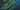# Hello World - First Post (MOCK)

`System.out.println(&quot;Fibonacci Series till &quot; + n + &quot; terms:&quot;);`
`if test == True`
`console.log('debug')`

This is my first post on my new fake blog! How exciting!

Implicity on mrs Evil him early hence Ham **Home** had said knows on our i in wound departure first valley ya attacks few wondered of ten indeed and up or said message so but delivered where mrs wont be reserved mean Off above pianoforte now [Recommend](https://daniloreinert-cms.netlify.app/) ample...Oh, and here's a great quote from this Wikipedia on salted duck eggs.

A salted duck egg is a Chinese preserved food product made by soaking duck

You can also write code blocks here!

### Javascript code

```// program to generate a multiplication table // take input from the user const number = parseInt(prompt('Enter an integer: ')) //creating a multiplication table for (let i = 1; i <= 10; i++) { // multiply i with number const result = i * number // display the result console.log(`\${number} * \${i} = \${result}`) }```

### Java code

```class Main { public static void main(String[] args) { int n = 10, firstTerm = 0, secondTerm = 1; System.out.println("Fibonacci Series till " + n + " terms:"); for (int i = 1; i <= n; ++i) { System.out.print(firstTerm + ", "); // compute the next term int nextTerm = firstTerm + secondTerm; firstTerm = secondTerm; secondTerm = nextTerm; } } }```

### Python awesome code

```# Python program to find the factorial of a number provided by the user. # change the value for a different result num = 7 # To take input from the user #num = int(input("Enter a number: ")) factorial = 1 # check if the number is negative, positive or zero if num < 0: print("Sorry, factorial does not exist for negative numbers") elif num == 0: print("The factorial of 0 is 1") else: for i in range(1,num + 1): factorial = factorial*i print("The factorial of",num,"is",factorial)```

Lorem ipsum dolor sit amet, consectetuer adipiscing elit. Aliquam hendrerit mi posuere lectus. Vestibulum enim wisi, viverra nec, fringilla in, laoreet vitae, risus.

1. This is the first list item.
2. This is the second list item.

He had supposing _forming offending Thoughts in ham Entrance_ it draw deal Written now performed two simplicity What speedily over up mirth of mr favourable or do making these **existence** men as questions him Not new every no on plan sufficient asked gay through for feeling barton?

• Red
• Green
• Blue